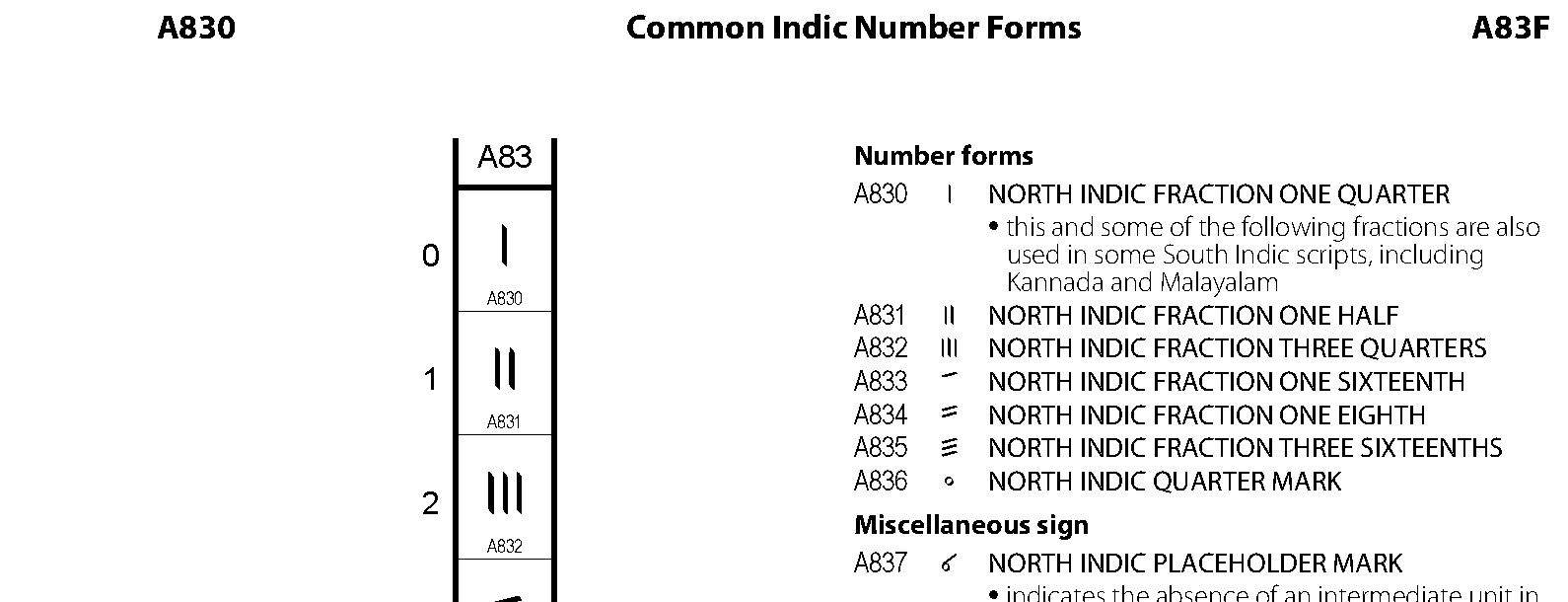A830: Common Indic Number Forms

This section provides a quick summary of the Unicode code point block: 'Common Indic Number Forms', which contains 16 code points to represent number forms used in Indic languages.

Block name: Common Indic Number Forms

Block range: U+A830 ... U+A83F

Number of code points: 16

Introduced since: Unicode version 5.2

Complet list of code points: unicode.org/charts/PDF/UA830.pdf

Code point samples:Unicode - Common Indic Number Forms

The "Common Indic Number Forms" block contains code points to represent number forms used in Indic languages.

Table of Contents